### Home > A2C > Chapter 12 > Lesson 12.5.1 > Problem12-200

12-200.
1. Use what you just proved about the sum and product of the roots of a quadratic equation, in problem 12-199, to write a quadratic equation for each of the following pairs of roots. Homework Help ✎

1.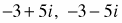2.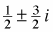3.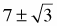4. 7, −6

5.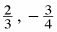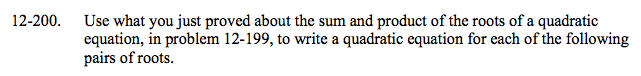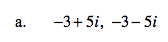$(-3 + 5i)+(-3-5i)=-\frac{b}{a}\rightarrow-6=-\frac{b}{a}$

$(-3+5i)(-3-5i)=\frac{c}{a}\rightarrow9-(-25)=\frac{c}{a}$

Choose a to be 1 and write the general quadratic equation using the values you find for b and c.

x2 + 6x + 34 = 0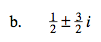See part (a).

x2x + 1 = 0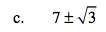See part (a).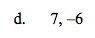See part (a).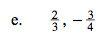See part (a).